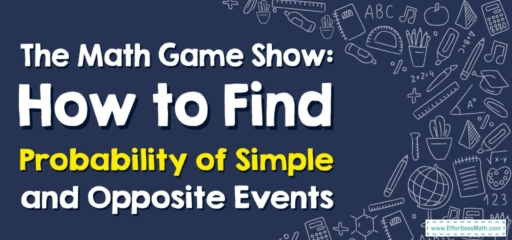# The Math Game Show: How to Find Probability of Simple and Opposite Events

Welcome, ladies and gentlemen, to the most exciting game show in the world of math - Probability Showdown!

In today's thrilling episode, we're diving into two very intriguing aspects of probability - Simple Events and Opposite Events. So, let's not wait any longer. Let the games begin!## 1. Today’s Contestants: Simple Events and Opposite Events

In the left corner, we have Simple Events – these are the events that consist of a single outcome. In the right corner, we have Opposite Events, also known as the complement of an event.

## 2. The Showdown: Probability Calculation

In this game show, understanding the probabilities of these contestants will help us anticipate their moves and strategies.

### Your Game Show Guide: How to Calculate Probability of Simple and Opposite Events

#### Step 1: Understanding Simple Events Probability

In any probability event, the likelihood of a simple event happening is calculated by dividing the number of desired outcomes by the total number of possible outcomes.

#### Step 2: Understanding Opposite Events Probability

Opposite event, or the complement of an event, is the event that the desired outcome does not occur. The probability of an opposite event is calculated by subtracting the probability of the desired event from $$1$$.

Let’s consider a simple example using a standard deck of $$52$$ cards.

If we want to calculate the probability of drawing a king (a simple event):

1. Understanding Simple Events Probability: There are $$4$$ kings in a deck of $$52$$ cards. So, the probability of drawing a king is $$4$$ (desired outcomes) divided by $$52$$ (total outcomes), which equals approximately $$0.077$$ or $$7.7$$$$\%$$.
2. Understanding Opposite Events Probability: The opposite event here is not drawing a king. So, the probability of not drawing a king is $$1$$ (total probability) minus $$0.077$$ (probability of drawing a king), which equals approximately $$0.923$$ or $$92.3$$$$\%$$.

And that’s it for today’s episode of Probability Showdown, folks! I hope you had as much fun exploring probabilities as we did. Remember, life is full of probabilities, and understanding them can make you a true game show champion. See you in the next episode!

### What people say about "The Math Game Show: How to Find Probability of Simple and Opposite Events - Effortless Math: We Help Students Learn to LOVE Mathematics"?

No one replied yet.

X
30% OFF

Limited time only!

Save Over 30%

SAVE $5 It was$16.99 now it is \$11.99